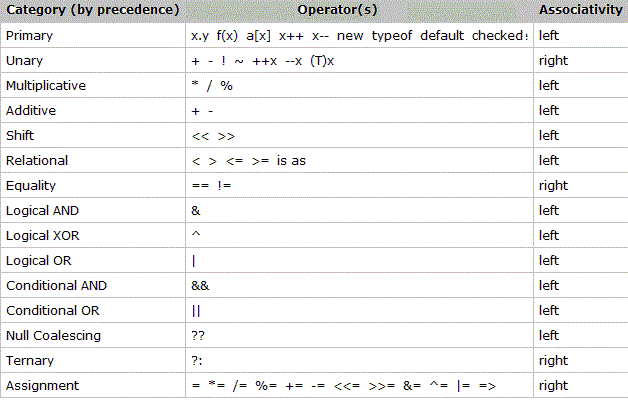Not Understanding Order of Operations With Multiple Types: Which Type Gets Priority?

int a = 3;
csharp> int b = 2;
csharp> double c = 3.5;
csharp> double y = a / b * c;

//The correct answer to the above is 3.5
//as we're to be executing a/b first and then that result divided by c

Just because I can get the right answer here doesn't mean I understand why this is the correct answer....What I'm not understanding here is two-fold:

1) Why, given that Multiplication and Division have equal standing in terms of execution order in math, is a/b executed first? In this answer: https://teamtreehouse.com/community/integers-and-doubles-question-7 @Pablo Rocha implies that integers have priority over floats (aka doubles)....or is it simply that the math based code here is "read" from left to right: a/b comes before b*c? (it was my understanding that C# is "read" by the computer from right to left with assignment...so it should have been "read" by the REPL as c * b/a, which would result in 2.33 repeating)

2) If it is the case that ints have priority somehow over doubles (aka floats) are there exceptions to this priority? What about the math order of operations? How do type priorities work with math order of operation priorities?Math evaluation (and its order) takes precedence over assignment evaluation.

• assignments are performed right to left meaning everything on the right of the "=" is evaluated first
• this does not affect the order of evaluation of the right-side terms themselves
• math operations (including order) take precedence over assignment operations
• math operations of equal priority are performed left to right
• types do not affect math evaluation order

For reference, this MSDN Page lists operators in order of precedence.

Steven Parker : Thanks for the start here! I'm wondering next about order of operations here. In math when you're faced with "equal ranking" operations (multiplication and division) you read from left to right to solve; however in C# the computer reads from right to left....so I'm not understanding if C# solves this using the C# programming logic or mathematical logic. Programming logic would dictate to me that you'd do this order: c * b / a which results in 2.33 but I know that's not what we get...which then implies that the computer switches to mathematical logic so a/b * c which results in 3.5 due to truncation. However, is this universal or is there times when "programming logic" as I'm calling it is applied first before mathematical logic?The computer does not "read right to left".

Each operator has it's own direction, and math operations are evaluated left-to-right. Assignment operations are evaluated right-to-left, but they have a much lower precedence than math operations.I found this little chart that might be helpful:Note that while assignments are right-to-left, they come last in precedence.